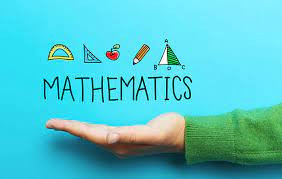Search

# Mathematics Project Help | Maths Projects - List of Math Project Models and Examples | Softcodershub

Updated: Jan 26

#MathematicsProjectHelp

#MathsProjectsListofMathProjectModelsandExamples

#Softcodershub

## Math projects -

Here is a downloadable PDF that consists of Math projects for Grades 6-10. Click on the download button to explore them. Understanding and grasping the ideas of mathematics in a better way.## These maths projects help in developing very important mathematical skills like:-

• Correlating the concepts taught in the classes with the practical applications of those concepts

• Proving a hand on experience to the children

• Fostering teamwork, coordination, and communication along with creativity and knowledge

• Understanding and grasping the ideas of mathematics in a better way

• Visualizing the concepts in the form of diagrams, graphs, and images facilitates a better understanding

• Improving their problem-solving skills, reasoning, and planning skills, etc.

• Making real-life decisions that leads to a holistic approach to learning.

## Maths project ideas for Class 6 To 10 -

Some of the ideas for simple maths projects for class 6 are:

1- Number System Tree -

The number system is one of the most basic concepts in mathematics. It is very important to understand the different types of numbers (order and even numbers, fractions and decimals, natural numbers, whole numbers, positive and negative numbers, etc.) and the different properties of numbers. A project for the same will help the student understand and correlate the relations between different types of numbers.

2- Shapes and Figures -

Shapes, figures, and sizes are a very important concept of geometry. A student must understand the different properties of different geometrical figures. It starts from class 6 but has various applications in higher-level education. Therefore it is very important to have a proper foundation that is only possible through a project on the same.

3- Practical examples of different chapters -

From class six onwards, students start getting exposed to different topics that have a number of practical applications like percentages, profit, and loss, interest, etc. Students can be asked to make their own problem statements by practicing creative problems with respect to the same chapters. This will help them to correlate the concepts with the practical world.

4- History of maths and different topics -

It is very important to understand the history or the background of a subject or a topic before starting to learn more about it. But, because of the curriculum norms, this very important aspect is skipped from the school syllabus. Projects can be a great way to help students do research about it. This will facilitate better knowledge and understanding.

5- Puzzles and brain teasers projects -

Puzzles and brain teasers are a very dynamic way of inculcating knowledge, fostering creativity, and facilitating practical viewpoints. This helps a student to think and come up with answers along with reasons to support it. It helps them in a better decision making process.

6- Algebraic expressions -

Students are exposed to a new field of mathematics in class 7 which is algebra. Good project work can help a student to develop a strong base in understanding different algebraic equations and expressions. This project work can include a number of numerical, interesting problem statements, and mental math calculations.

7- Practical examples of different chapters -

This project work is actually never-ending. Students don't learn all the concepts in a single standard. They continue learning various different concepts in different chapters that have different practical applications. Slowly, the level of difficulty keeps on increasing. Therefore it is very important to stay updated and improvise the skills through project works which allow us to correlate the concepts of the chapters with practical examples.

8- Mensuration of figures -

For the first time in class VIII, a student gets exposed to the chapter on menstruation. This chapter facilitates measurements of different things. It may include length, perimeter, area, etc. There are a number of concepts and a number of formulas that are related to this particular topic. Therefore giving good project work that helps students to understand these concepts by applying the given formulas and correlating them with the practical ships will foster growth and development in knowledge.

9- Heights and Distances -

A student gets exposed to the concept of trigonometry for the first time in class 9-10. This concept is very widely used to understand heights and distances which plays a very important role in practical life. This also develops a base for various higher-level studies. Students can be asked to draw diagrams and graphs and correlate the concepts with the same to develop a better understanding.

10- Statistics and graphs -

It is in class 9 that a very new dimension of mathematics opens up for the students which are known as statistics. A very important part of statistics is graphical representations that have their application in almost every sphere of knowledge. Therefore, it becomes very important to understand the basics of these concepts and good project work on this topic will definitely foster good learning.

11- Making and understanding 3D figures -

There are various 3-D figures which a student must understand, like cubes and cuboid being the basics. The calculations for these 3-D figures are not as easy as the 2-D figures. Therefore, good project work will facilitate the visualization of 3-D figures and also help students to understand the various formulas and calculations related to it.

12- Similarity and Congruency -

Another very important concept of geometry that pops up in class 9-10 is the similarity and congruency of triangles. Students often face a lot of difficulty in understanding these concepts. Therefore, a project work that has a good amount of research work with a number of assignments and questions to solve will definitely help a student to learn the concept of similarity incongruency.